## Introduction

The presence of topological order in matter is responsible for fundamental phenomena, such as the fractional and integer quantum Hall effects1,2 or the protected surface states observed in topological insulators3,4. Non-trivial topological phases are related to symmetries and can be characterized by specific topological invariants, defined in terms of the energy band eigenstates. Besides physical systems that naturally exhibit such features, the same topological scenarios have been reproduced in easy-to-access artificial systems (quantum simulators), which represent convenient platforms for the investigation of the intriguing properties associated with these states of matter5,6,7,8,9. In particular, a variety of simulators based on photonic architectures have been realized10,11,12,13,14,15,16.

In the field of quantum simulation of topological phenomena, quantum walks (QWs) are emerging as a versatile tool17,18,19. In its simplest version, a QW is the discrete-time evolution of a particle (the walker) on a one-dimensional (1D) lattice20. At each step, the walker can move to either one of the two nearest-neighbour sites of the lattice, as determined by the configuration of an internal two-state quantum system (the coin). Between consecutive steps, the coin state undergoes a rotation that determines the relative probability amplitudes of the subsequent walker move, thus providing the quantum version of the random choice process (the coin toss) characterizing the familiar classical random walk. Among simulators of topological physics, QWs are attracting a wide attention since this simple quantum dynamics can realize all topological phases occurring in one- and two-dimensional systems of non interacting particles18; as a first application of this concept, the formation of topologically protected bound states was observed in a photonic QW10.

In this paper, we report the study of a QW process that exhibits two distinct topological phases, associated with chiral symmetry in a 1D bipartite lattice. Remarkably, identical symmetries and topological features characterize the Su–Schrieffer–Heeger model (SSH), describing for example the mobile electron dynamics in the poly-acetylene chain21, and the effective theory for spin-less 1D superconductors showing p-wave pairing22. By studying the QW protocol introduced here, we found that the probability distribution moments for the particle position, in the limit of an infinitely long temporal evolution, show a different asymptotic behaviour for the two topological phases: varying an external control parameter, whose value determines the phase of the system, they exhibit a slope discontinuity at the quantum transition. These effects are found to occur also in other 1D topological systems, including models belonging to different topological classes; hence, we conjecture here that they are fully general in 1D. We simulated experimentally such phenomena and proposed a theoretical interpretation based on the dispersion relations and the geometric features of the system eigenstates. Remarkably, our analysis takes into account bulk dynamics only, and not the physical effects manifesting at the edges of the system. With a similar approach topological phases have been recently detected in a non-Hermitian QW,17,11 while all the models we investigate here are Hermitian.

## Results

### Quantum-walk protocol based on light spin–orbit interaction

In recent years, QWs have been implemented in a variety of physical architectures23. In our photonic platform24, the walker is encoded in the orbital angular momentum (OAM) of light25: discrete positions on the lattice are associated with states |m〉, where m is an integer, describing a photon carrying of OAM along its propagation axis. The coin is encoded in the polarization degree of freedom, that is, in the spin angular momentum (SAM) of photons: vectors |L〉 and |R〉, representing left and right circular polarizations (SAM of ±ħ per photon), respectively, are the two internal states that determine opposite shift directions in the lattice. The quantum state of the photon after N steps of QW is denoted as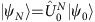, whereis the initial (input) state and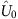is the unitary evolution operator of a single QW step. In our protocol, this is realized by cascading two optical elements: a quarter-wave plate (QWP) and a q-plate (QP). A q-plate is essentially a liquid-crystal birefringent cell having the optic axis arranged in a singular pattern26, with topological charge q (in our case q=1/2). This patterned birefringence gives rise to an engineered optical spin–orbit coupling that induces the polarization-controlled shift of OAM. As specified below (see equation 2), besides the charge q, the action of this device is determined by the value of the optical retardation δ, which can be tuned with an applied electric field27; this allows in turn for a fine control of the light spin–orbit interaction mediated by the plate. In our protocol, the QWP is oriented at 90° with respect to the horizontal direction; its action is described by the operator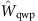, that transforms the polarization states as follows: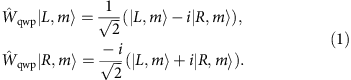The q-plate action is described by the operator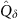, defined as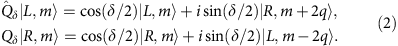Here we can observe that the spin–orbit interaction introduced by the plate, proportional to sin(δ/2), results in an exchange of angular momenta, that is a conversion from SAM to OAM. The combination ofandrealizes the operator for a single QW step, that is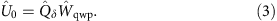A topological characterization of QWs with chiral symmetry may be defined in terms of the eigenstates of the operatorwhich, due to the lattice translation symmetry, are associated with two quasi-energy bands parameterized by a quasi-momentum k, as shown in Fig. 1a. As for momentum k, a periodic quasi-energy variable E replaces here the usual energy, being the time (= step number) a discrete variable. Accordingly, the dispersion relation for our QW is given by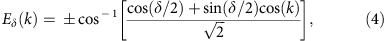where the two signs correspond to the two bands. As a consequence, the expression of the associated group velocity is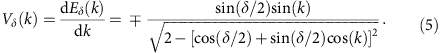The band eigenstates are given by the product of a walker part, that is plane waves with quasi-momentum k in the walker space, and a coin part, denoted as |φs,δ(k)〉, where s{1, 2} is the band index. Conveniently, these states can be represented as points on the Poincaré sphere for light polarization, individuated by a three-dimensional unit vector that we refer to as nδ(k); here we report the explicit expressions of its components, that is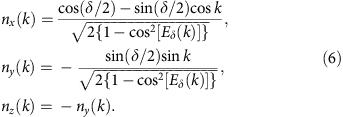Chiral symmetry constrains vectors nδ(k) to lie on a great circle of the sphere. When k varies throughout the Brillouin zone {−π, π}, the number of closed loops (winding number) described by nδ(k) is a topological invariant, labelled here as W. As shown in Fig. 1c the value of δ in the range {0, 2π}, that is the strength of the spin–orbit interaction, determines the existence of two phases with a different winding number: a phase with W=2q=1 occurs when δ1<δ<δ2, while W=0 in the remaining regions. As pointed out in refs 19, 28, for time-periodic systems with chiral symmetry a complete topological classification would require introducing two topological invariants, so W alone is not enough. Nevertheless, this is not necessary for the purposes of this work, as we focus our attention on the phase transitions only, while we do not need to identify completely the nature of the topological phases, and it can be shown that all topological phase transitions are associated with a change of W19,28. Therefore, to keep the discussion simpler we will use only the winding number W in our discussion. In this sense, we will refer to the phases W=0 and 1 as trivial and non-trivial phases, respectively. In Fig. 1a it can be noted that when δ=δ1 and δ=δ2 the two bands are touching and the dispersion is locally linear at k=0 and k=π, respectively, as typically occurs for quantum transitions between topological phases3. Interestingly, comparing the expressions of group velocity Vδ=dEδ/dk (equation 5) and vector nδ(k) (equation 6), we can observe that nz=−ny=|Vδ|. Thus in the non-trivial phase, where the trajectory of nδ(k) is fixed, the maximum of the group velocity is independent of δ (see the Supplementary Note 1 and the Supplementary Fig.1 for further details). A recently introduced QW protocol, that is the split-step QW, has very similar properties10,18.

### Dynamical moments and topological phases

The features of the energy bands and associated eigenstates have profound consequences on the system dynamics, which shows marked differences in the two topological phases11,17. Here we characterize such different behaviour through the analysis of the moments of the probability distribution P(m) associated with the walker position, defined as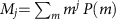. In particular, we consider a photon starting its walk in the position m=0 with an arbitrary polarization, that is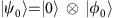, where |φ0〉 is a generic state in the coin Hilbert space. Simulations of the QW evolution in the large step-number limit show that the moments Mj assume a constant value (independent of δ) in the non-trivial phase, and undergo abrupt slope variations at the phase transitions, that is at δ={δ1, δ2}. In the infinite-steps-limit, we proved that these moments have simple asymptotic expressions in terms of the energy band dispersion relations; in particular, those relative to the first and second moments M1 and M2 are the following (see Methods for a proof):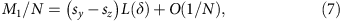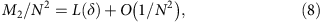where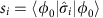, with i{x, y, z}, are the reduced Stokes parameters, calculated as the expectation values of the Pauli operators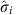for the coin initial state |φ0〉. Interestingly, we observe that M2 is independent of the initial coin state. The quantity L(δ) appearing in equations 7 and 8 is equal to the square of the group velocity Vδ, and hence to the square of ny, averaged over the Brillouin zone: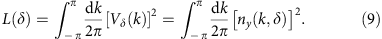For our QW process, the integral in equation 9 admits a closed form, that is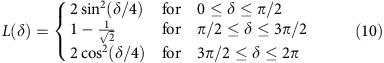In Fig. 2a–c, this expression is plotted as a function of δ; as a consequence of the discontinuity present in the group velocity, L(δ) is a piecewise function, and manifests abrupt slope variations at δ1=π/2 and δ2=3π/2. As shown in Fig. 3 and thoroughly discussed in the Supplementary Note 2 and in the Supplementary Figs 2–6, by applying a similar approach to other 1D topological systems we were able to observe very similar features. For our QW, in the non-trivial phase L(δ) is constant; this result can be qualitatively understood by looking at how the dispersion law of the group velocity is modified by a change of δ (Supplementary Fig. 1). On the other hand, in the trivial phase L(δ) increases or decreases in the two regions {0, π/2} and {3π/2, 2π}, respectively. Relying on a numerical analysis, we find that such features are robust to the presence of disorder arising from possible experimental imperfections in our setup (details are provided in Methods and in Supplementary Fig. 7). In a QW with a finite number of steps, statistical moments M1 and M2 have a continuous behaviour, converging to that given by equations 7 and 8 asymptotically as N→∞. For M2, this convergence is rapid and visible for values of N that are small enough to be achieved in an experimental simulation, and it is independent of the coin initial state, whereas for M1 such process is much slower (see Fig. 2). Thus, as an indicator for identifying the quantum transitions, we chose to use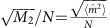, corresponding to the width of the OAM statistical distribution, normalized to the number of steps. In particular, we implemented a six-step QW and measured the OAM distribution width when tuning the spin–orbit interaction in each q-plate, that is δ, so as to realize the topological quantum transition.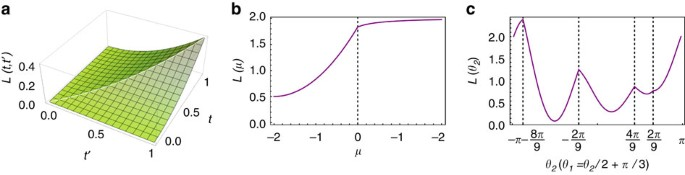Figure 3: Dynamical moments reveal topological phase transitions in other 1D topological systems.

### Experimental results

The layout of the apparatus is shown in Fig. 4. A standard heralded single-photon source is realized at the input of the QW system, as discussed in Methods. Before undergoing the QW evolution, a single photon is prepared in localized initial state (m=0), with its polarization (the coin part) described by |φ0〉=α|L〉+β|R〉. The value of the two complex coefficients α and β (with |α|2+|β|2=1) can be tuned by suitably orienting two waveplates that are positioned at input of the setup (see the caption of Fig. 4 for more details). After the QW dynamics, the photon state is analysed in both polarization and OAM so as to determine the output probabilities and the associated moments. Letting δ vary in the range {0, π} with steps of π/16, we determined the corresponding probability distribution of the walker after a six-step QW; a typical example is reported in Fig. 5a. In Fig. 5b,c we plot the measured values for M1/N and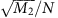, respectively, as a function of δ. Experimental data match well the quantum predictions and, as expected, they are close to the asymptotic limit reported in equations 7 and 8 only in the case of. For the latter, the emergence of an abrupt slope variation at δ=π/2 can be appreciated; the observed non-analyticity is a signature of the underlying quantum transition. In addition, M2 is observed to be locked to a constant value in the non-trivial phase. On one hand, this result reflects the features of the group velocity and the strong link between the latter and the geometry and topology of the system eigenstates. On the other hand, this is not a general feature of 1D topological systems; in the Supplementary Note 2 we show that for other models, such as the SSH, this plateau can be observed in either of the two phases, depending on the specific trajectory followed in the parameters space. Yet, it is possible to show that for our QW (equation 9) and the SSH model (Supplementary Note 3), the value of M2 is proportional to the integral of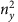over the Brillouin zone and that the latter always shows a constant value, that is a plateau, when W=1. Preparing the initial state of the coin (m=0 for the walker) in two non-orthogonal polarizations we also verified that M2 (unlike M1) is independent of the coin initial conditions, as expected from equation 8 (see Fig. 5b,c). This aspect was further investigated by measuringfor several initial polarizations, corresponding to specific points along a meridian of the Poincaré sphere, and repeating the experiment for two values of δ in each topological sector. The final data, reported in Fig. 5d, match well the predicted results and clearly show that the value of the second-order moment is independent of the coin initial state; this guarantees the robustness of our results with respect to imperfections in the initial state preparation.

## Discussion

The main result of this work is the idea that a signature of quantum transitions between distinct topological phases is present in the behaviour of suitable dynamical observables that are easily accessed experimentally. In the context of 1D topological systems, we propose that the statistical moments of the particle probability distribution in space (in the large step-number limit) provide a convenient choice of such observables. We experimentally validated this proposal by simulating this topological environment within a specific photonic QW architecture. The measured asymptotic moments as a function of a control parameter show slope discontinuities at the phase change, and hence can be used as direct indicators of the phase change occurrence. It is remarkable that these observables reflect bulk properties only, in contrast with the common strategy that relies on investigating topological physics exclusively through edge effects. Numerical simulations show that these features are robust to experimental imperfections and confirm their presence in several other 1D topological systems, thus strongly supporting the conjecture of their general validity in 1D. Moreover, we are currently investigating the possibility to apply similar concepts to 2D systems. Localization induced by spatial disorder is of course expected to affect the final asymptotic behaviour of the dynamics. However, if the disorder is not very strong, it is plausible that an intermediate time regime will still exist, in which the quasi-nonanalytic behaviour of the moments can be detected. In prospect, our approach based on dynamical moments could be applicable to the investigation and to the detection of other classes of quantum transitions, and to topological phases associated with more complex symmetries or with a higher dimensionality, thus helping to shed new light on the physics of topological phenomena and on their simulation in suitable experimental architectures.

## Methods

### Asymptotic expressions of first and second moments

The large step-number limit for the walker distribution moments (equations 7 and 8) can be derived conveniently by evaluating M1 and M2 in momentum representation, where they are defined as follows: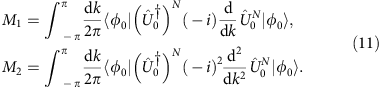Here N is the step-number,is the single-step evolution operator (3), and |φ0〉 is the coin initial state. Expanding the evolution operator aswhere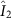is the identity matrix in 2D,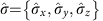and the components of nδ(k) are those reported in equation 6, it is straightforward to obtain the following equations: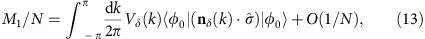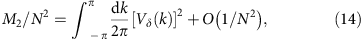It is important to observe that equations 13 and 14 are valid for any quantum system whose Hilbert space has the same structure as our QW, that is the direct product of an infinite discrete lattice and a two-state quantum degree of freedom. Equation 13, reporting the expression for the first-order moment, can be evaluated considering that Vδ=nz=−ny. Applying this substitution, the same equations reads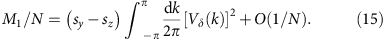First- and second-order moments reported in equations 14 and 15, respectively, coincide with the equations 7 and 8 of the main text. In the specific case of our QW model, the integral appearing in equations 14 and 15 can be solved analytically. Using the expression for the group velocity reported in equations 5 and 9 yieldsThis integral can be calculated by the residue theorem passing to the complex variable z=eik. Then we obtain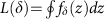, where the integral is along the unit circle in the complex z-plane and fδ(z) is given by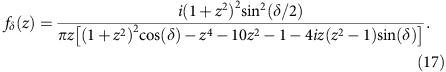The poles of fδ(z) are located on the imaginary axis at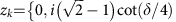,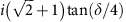,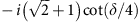and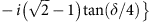(k=1, … 5) and the residues of fδ(z) at the poles are given by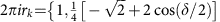,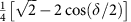,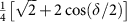and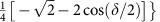, respectively. Apart from the pole at z=0, when δ varies, the locations of the poles move in the complex plane entering and exiting the unit circle, but only the residues of the poles inside the unit circle contribute to L(δ). Thus, we find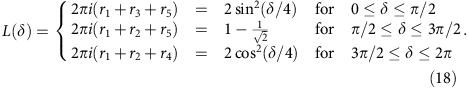In Supplementary Note 3 and in Supplementary Fig. 6 we show how with the same approach the analytical expression for the function L can be found for the SSH model.

### Heralded single-photon source

In the heralded single-photon source (the optical components used to realize this source are not shown in the setup scheme of Fig. 4, for details see ref. 24), laser pulses (100 fs) at 800 nm generated by a titanium–sapphire source (Ti:Sa) with repetition rate 82 MHz shine on a type-I β-barium borate crystal for second harmonic generation; frequency-doubled pulses at 400 nm, with 110 mW average power and linear-horizontal polarization, pump a type-II β-barium borate crystal, cut for collinear and degenerate spontaneous parametric down-conversion. Signal and idler photons, generated in horizontal and vertical linear polarizations, respectively, are spatially separated by means of a polarizing beam-splitter and then coupled into single-mode fibres; the idler photon is directly sent to an avalanche photodiode (APD D1), while the signal one is sent to the QW system. After the QW evolution, such photon is analysed in polarization and OAM and finally detected by APD D2, in coincidence with D1. In this way, the idler photon is used as a trigger, which heralds the presence of the correlated particle passing through the QW setup.

### Experimental imperfections and role of disorder

Experimental realizations of quantum protocols and quantum dynamics exploit specific devices whose behaviour may present deviations with respect to their ideal features. Here we individuate possible imperfections that may arise in our system, discussing in turn how they would affect our QW protocol and, as a consequence, the dynamical moments associated with the walker wavefunction. Interestingly, we find that abrupt variations at the phase change are robust to these kinds of disorder.

A first type of imperfection can result from a bad orientation of the QWPs performing the coin rotation. We considered two different coin input, namely |H〉 and |R〉 polarizations already used in the experiment, and simulated a 80 steps QW, where at each step a QWP is oriented at ±2° with respect to the correct angle; here we assumed that at 0° a QWP implements the operator reported in equation 1. The ± sign is randomly selected. In Supplementary Fig. 7, we report the results of our numerical analysis. Data clearly show that the second-order moment have no significant differences with respect to the ideal QW; accordingly the phase change can be appreciated still, thus proving the robustness of such indicator. For |R〉 as initial state, the mean value has a similar behaviour, whereas something different happens for the |H〉 polarization; in the latter case, ideal QW leads to a vanishing mean value. When QWPs have random bad orientations, this is not true anymore, even though deviations from ideal case are smaller than typical experimental errors affecting our data. A second source of errors can arise from the bad alignment of q-plates. In particular, their centre could be displaced with respect to the propagation axis of the beam. As a first approximation, the q-plate operator reported in equation 2 would be transformed as follows: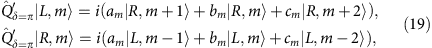where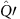is the operator describing the q-plate action when a displacement of its centre is taken into account; here it has been considered the simple case δ=π (the term proportional to cos(δ/2) is the identity operator, which is not affected by such displacement). Moreover, we are explicitly neglecting the coupling to spatial modes of light with different radial distributions, as for instance Laguerre–Gauss modes with radial index p≥1. We can note that in equation 19 non-vanishing values for cm are responsible for next-nearest-neighbour couplings in the OAM lattice, corresponding to an ‘off-diagonal’ disorder29. Coefficients {am, bm, cm} can be computed numerically; interestingly, the effects of the q-plate displacement are not equal for all OAM states; in particular, deviations from the ideal case becomes negligible for OAM modes with high |m|; for such states, am1 and bmcm0. Similarly to the previous analysis we implemented a numerical simulation of a 80 steps QW for |H〉 and |R〉 coin input; at each step the q-plate is displaced randomly along the x or the y axis (the z axis corresponds to the propagation direction of the light beam). We numerically computed coefficients {am, bm, cm}, assuming a displacement equal to 5% with respect to the beam transverse dimensions (that is the radius at the waist of the input Gaussian beam). Data reported in Supplementary Fig. 7 show that the effect of such imperfections is negligible. Importantly, the abrupt variation at the phase change can be still appreciated, thus proving the robustness of such features. Even for this case the mean value for |H〉 has no significant differences when compared with the ideal case, being it very close to zero for all values of δ (within our experimental uncertainties). In conclusion, we want to compare such imperfections with the presence of static disorder, which is typically responsible of localization phenomena. An imperfect orientation of the waveplates leads to a modification of the coin operator that is equal for all OAM states, thus it cannot produce any localization. Effects associated with the q-plate displacement are not uniform in the OAM space, but still they are quite different with respect to the typical static disorder, that consists in random site-dependent phase shifts, equal at each step. Moreover, in both cases alterations of the step operator are different at every step of the walk, thus they represent a temporal disorder. Importantly, our simulations show the robustness of moments non-analyticities as indicators of quantum phase changes in such disordered scenarios. Although the spatial static disorder is certainly not significant in our experimental implementation, it can be obviously important for the generalization of our results to other systems. Localization induced by this kind of disorder is expected to affect the final asymptotic behaviour of the dynamics. However, if the disorder is not very strong, it is plausible that an intermediate time regime will still exist in which a quasi-nonanalytic behaviour of the moments can be detected. If ‘localization time’ is sufficiently larger than the measurement time, the disorder will not affect the kinks (slightly rounded in real experiments because of the finite measurement time) that signal the occurrence of the topological transitions. Hence, we believe that our main results on the use of moments for detecting topological transitions can be useful also in the context of disordered systems, as long as the disorder is not too strong.